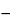Levocarnitine
(lee'' voe kar' ni teen).

C7H15NO3161.20
(R)-3-Carboxy-2-hydroxy-N,N,N-trimethyl-1-propanaminium, inner salt;
(R)-(3-Carboxy-2-hydroxypropyl)trimethylammonium, inner salt[541-15-1].
DEFINITION
Levocarnitine contains NLT 97.0% and NMT 103.0% of levocarnitine (C7H15NO3), calculated on the anhydrous basis.
IDENTIFICATION
Analysis: Dry the sample and the USP Levocarnitine RS under vacuum at 50for 5 h.
Acceptance criteria: Meets the requirements
ASSAY
•  Procedure
Sample: 100 mg of Levocarnitine
Blank: A mixture of 3 mL of formic acid and 50 mL of glacial acetic acid
Titrimetric system
Mode: Direct titration
Titrant: 0.1 N perchloric acid VS
Endpoint detection: Visual
Analysis: Dissolve the Sample in a mixture of 3 mL of formic acid and 50 mL of glacial acetic acid. Add 2 drops of crystal violet TS, and titrate with the Titrant to an emerald green endpoint. Perform the Blank determination.
Calculate the percentage of levocarnitine (C7H15NO3) in the portion of Levocarnitine taken:
Result = {[(VSVB) × N × F ]/W} × 100
 VS = = Titrant volume consumed by the Sample (mL) VB = = Titrant volume consumed by the Blank (mL) N = = actual normalilty of the Titrant (mEq/mL) F = = equivalency factor, 161.2 mg/mEq W = = Sample weight (mg)
Acceptance criteria: 97.0%–103.0% on the anhydrous basis
IMPURITIES
Standard: 0.50 mL of 0.020 N hydrochloric acid
Sample: 0.090 g of Levocarnitine
Acceptance criteria: NMT 0.4%
•  Limit of Potassium
[Note—The Standard solution and the Sample solutions may be modified, if necessary, to obtain solutions of suitable concentrations adaptable to the linear or working range of the instrument. ]
Standard solution: 31.25 µg/mL of potassium in water, prepared from potassium chloride, previously dried at 105for 2 h
Sample stock solution: 0.625 mg/mL of Levocarnitine in water
Sample solution A: Transfer 20.0 mL of the Sample stock solution to a 25-mL volumetric flask, and dilute with water to volume. This solution contains 500 µg/mL of Levocarnitine and 0 µg/mL of added potassium from the Standard solution.
Sample solution B: Transfer 20.0 mL of the Sample stock solution to a 25-mL volumetric flask, add 2.0 mL of the Standard solution, and dilute with water to volume. This solution contains 500 µg/mL of Levocarnitine and 2.5 µg/mL of added potassium from the Standard solution.
Sample solution C: Transfer 20.0 mL of the Sample stock solution to a 25-mL volumetric flask, add 4.0 mL of the Standard solution, and dilute with water to volume. This solution contains 500 µg/mL of Levocarnitine and 5.0 µg/mL of added potassium from the Standard solution.
Blank: Water
Instrumental conditions
Mode: Atomic absorption spectrophotometry
Analytical wavelength: 766.7 nm
Lamp: Potassium hollow-cathode
Flame: Air–acetylene
Analysis
Samples: Sample solution A, Sample solution B, Sample solution C, and Blank
Determine the absorbances of the solutions against the Blank. Plot the absorbances of the three Sample solutions versus their added potassium concentrations, in µg/mL. Draw the straight line best fitting the three points, and extrapolate the line until it intercepts the concentration axis. From the intercept determine the concentration, in µg/mL, of potassium in Sample solution A.
Calculate the percentage of potassium in the portion of Levocarnitine taken:
Result = (CK/CU) × 100
 CK = = concentration of potassium in Sample solution A (µg/mL), determined from the intercept of the linear regression line CU = = concentration of Levocarnitine in Sample solution A (µg/mL)
Acceptance criteria: NMT 0.2%
•  Limit of Sodium
[Note—The Standard solution and the Sample solutions may be modified, if necessary, to obtain solutions of suitable concentrations adaptable to the linear or working range of the instrument. ]
Standard solution: 250 µg/mL of sodium in water, prepared from sodium chloride, previously dried at 105for 2 h
Sample stock solution: 40.0 mg/mL of Levocarnitine in water
Sample solution A: Transfer 20.0 mL of the Sample stock solution to a 25-mL volumetric flask, and dilute with water to volume. This solution contains 32 mg/mL of Levocarnitine and 0 µg/mL of added sodium from the Standard solution.
Sample solution B: Transfer 20.0 mL of the Sample stock solution to a 25-mL volumetric flask, add 2.0 mL of the Standard solution, and dilute with water to volume. This solution contains 32 mg/mL of Levocarnitine and 20 µg/mL of added sodium from the Standard solution.
Sample solution C: Transfer 20.0 mL of the Sample stock solution to a 25-mL volumetric flask, add 4.0 mL of the Standard solution, and dilute with water to volume. This solution contains 32 mg/mL of Levocarnitine and 40 µg/mL of added sodium from the Standard solution.
Blank: Water
Instrumental conditions
Mode: Atomic absorption spectrophotometry
Analytical wavelength: 589.0 nm
Lamp: Sodium hollow-cathode
Flame: Air–acetylene
Analysis
Samples: Sample solution A, Sample solution B, Sample solution C, and Blank
Determine the absorbances of the solutions against the Blank. Plot the absorbances of the three Sample solutions versus their added sodium concentrations, in µg/mL. Draw the straight line best fitting the three points, and extrapolate the line until it intercepts the concentration axis. From the intercept determine the concentration, in µg/mL, of sodium in Sample solution A.
Calculate the percentage of sodium in the portion of Levocarnitine taken:
Result = (CNa/CU) × 100
 CNa = = concentration of sodium in Sample solution A (µg/mL), determined from the intercept of the linear regression line CU = = concentration of Levocarnitine in Sample solution A (µg/mL)
Acceptance criteria: NMT 0.1%
SPECIFIC TESTS
Sample solution: 100 mg/mL in water
Acceptance criteria:29to32Sample solution: 50 mg/mL solution
Acceptance criteria: 5.5–9.5